## Confidence Interval for the population mean, μ, based on the sample mean when σ is unknown

Review:
The concept of a confidence interval was developed on a previous page. There we saw that we can take the sample mean,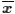, as a point estimate of a population mean, and form an interval with the point estimate as the center of the interval and the lower and upper ends of the interval set at a distance, called the margin of error, on either side of the point estimate. Furthermore, we used the fact that the distribution of sample means is normal to help us determine the value of the margin of error.

Part of that determination was to state the level of confidence that we want in the process. Thus, we create a 95% confidence interval by a process that will generate confidence intervals wide enough so that 95% of the confidence intervals generated by this process will contain the true population mean, μ.

To do this we used that the fact that the distribution of the sample mean is not only normal but it has a mean of μ and a standard deviation =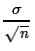. That meant that for a 95% confidence interval we needed to find a z-score,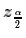, such that 95% of the area under the standard normal curve is between the z-score and its negative. Once we have the z-score then the margin of error is just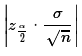and the confidence interval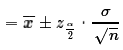.

New:
That whole process depends upon us knowing the value of σ, the population standard deviation. I hope that you can see how unlikely this is. The whole process is designed to find a confidence interval for the population mean. It would be absurd to do this if we already know the population mean. The process above requires that we know the population standard deviation. Since the population standard deviation is the root mean squared deviation from the mean, we need to know the population mean in order to compute the population standard deviation. It does raise the question of why we developed a process that we will never use?

We introduce the process above because it is a great model for creating a confidence interval when the population standard deviation is unknown. In such a situation we use the sample standard deviation, sx, as a approximation to the population standard deviation. When we do this can no longer compute the standard deviation of the sample mean bybecause we do not know σ. Instead, we compute the standard deviation of the sample mean by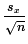. However, computed this way, we cannot say that the sample mean is N(μ,sx), instead we say that the sample mean has a Student's t distribution with n-1 degrees of freedom. Knowing this we can just mimic the earlier process, substituting the use of the Student's t with the appropriate degrees of freedom for ourvalue.

From a sample of size n=25 with a sample mean=134 and sx=6, to find a 95% confidence interval we look at the Student's t distribution for 25-1 = 24 degrees of freedom to find the t-score such that the area under the curve between that t-score and its opposite is 0.95 square units, (95% of the area). To do this we could look at the 24 degrees of freedom table to find the value that has 0.025 to its left. The portion of that chart that we would use is shown in Figure 1.

Figure 1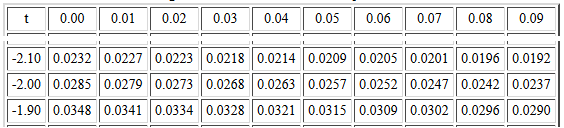The t-score -2.06 has 0.0252 square units to its left. The t-score -2.07 has 0.0247 square units to its left. We want to move 0.0002 of the 0.0005 square unit difference, that is, 2/5 of the way. Therefore, by interpolation, our best guess at the answer is -2.064.

Or, because we want the convenient 0.025 area to the left of the t-score, we could use the Critical Values of the Student's t table. The portion of that chart that we would use is shown in Figure 2.

Figure 2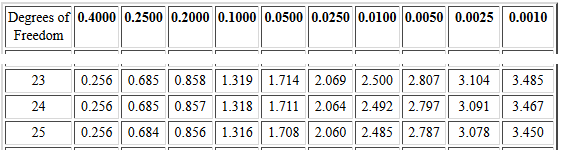There, on the row for 24 degrees of freedom and in the 0.0250 column, we find that the t-score 2.064 has 0.025 square units to its right. Note that this time we found the positive part of the pair of t-scores.

Or, because we can use R, we could just give either one of the two commands qt(0.025,24) or qt(0.025,24,lower.tail=FALSE), to find the t-score with 0.025 to the left or the t-score with 0.025 to the right, respectively. Figure 3 shows the commands and the results.

Figure 3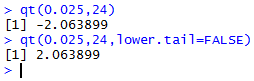It makes no difference if we find the positive or the negative t-score. The margin of error will be m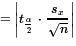and the absolute value means that the sign of the t-score does not make a difference in the result. When we compute the confidence interval the formula is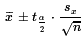and the ± means that the sign of the t-score will not make a difference.

For the problem we have been using, n=25,=134, sx=6, find a 95% confidence interval, the calculation of the margin of error and the resulting lower and upper endpoints of the confidence interval are shown in Figure 4.

Figure 4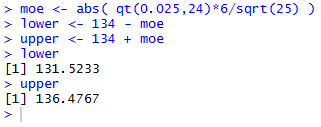Just as we saw in the case where we know the population standard deviation, we can change the magnitude of the margin of error in two ways. First, if we increase the level of confidence then the resulting confidence interval will be wider so that more of the samples produced using this process will include the true population mean. Alternatively, if we decrease the level of confidence then the resulting confidence interval will be narrower because we can have more of the intervals produced by this process miss the true population mean.

To illustrate this, we could do the same computations but for a 99% confidence interval (should be wider) and a 90% confidence interval (should be narrower). These are done in Figure 5.

Figure 5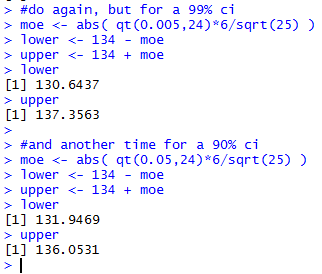The second way we can alter the width of the confidence interval is by changing the sample size. The larger the sample size the larger is the denominator in the formula for the margin of error resulting in a smaller value. If we return to the original problem, the 95% confidence interval but we increase the sample size to 70 then we will find that the resulting confidence interval will be narrower. That computation is shown in Figure 6.

Figure 6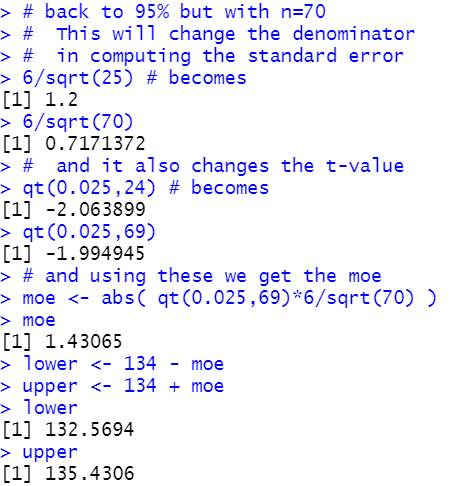If we have a desired margin of error, m, then we can use a little algebra to change m=into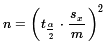to create a formula for determining the required sample size, n, once we have set the confidence level and we have a good idea about the eventual magnitude of sx. Note that each time we take a sample we will get a different sx but those values should not wonder around too much, especially for larger values of n. Thus, although we can get a good idea of the required sample size, we cannot get the exact value that we were able to get in the case where we knew the population standard deviation.

For the current example, where have a sample size of 25, we found a margin of error of about 2.4767. We could compute the sample size needed to get that down to 2.00 as shown in Figure 7.

Figure 7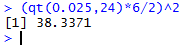That computation suggests that a sample size of 39 will be large enough to accomplish our goal. However, when we draw a sample of size 39 there is no way to be sure that the sample standard deviation, sx, will stay at 6.

This would be a good time to return to the web page that takes 1000 samples of a given size and computes confidence intervals for each sample. The starting web page is Set Parameters. Figure 8 shows the initial screen settings on that page.

Figure 8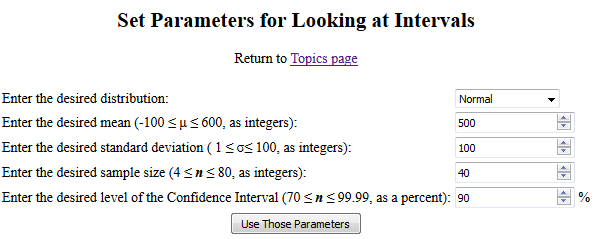We can change those values so that we are looking at a normal population with μ=134 and σ=6. Then we specify that we want samples of size 25 and we want to see 95% confidence intervals. Now the page appears as in Figure 9.

Figure 9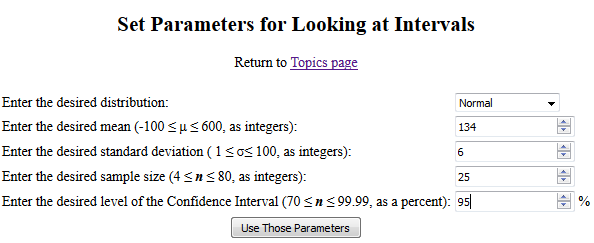At that point if we click on "Use Those Parameters" then we get a new page with a population, 1000 samples, and confidence intervals for each. Furthermore, each that you return to that page and click the button you get a new population and new samples. I clicked on the button and got a new page that I then saved so that it would be available, as a static page, for us to review. The page is at Saved Example.

That page starts with a confirmation of the desired population parameters and the listing of the population values. Figure 10 shows the parameters and first 20 values.

Figure 10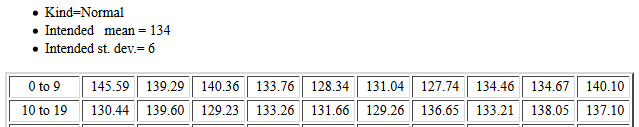Figure 11 shows the end of the population values and a statement that we indeed have met the expectations.

Figure 11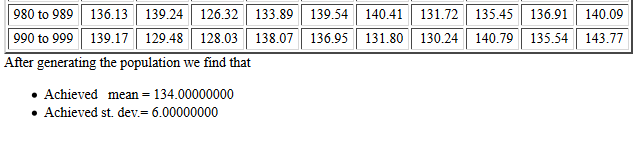The histogram of the population confirms the normal distribution characteristic.

Figure 12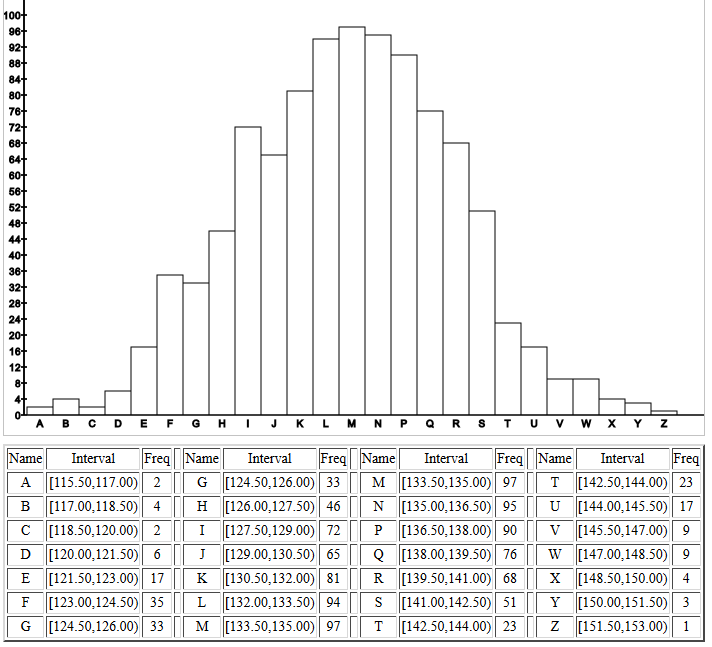Then, in Figure 13, we see mean and standard deviation of the first 30 of the 1000 samples, each of size 25.

Figure 13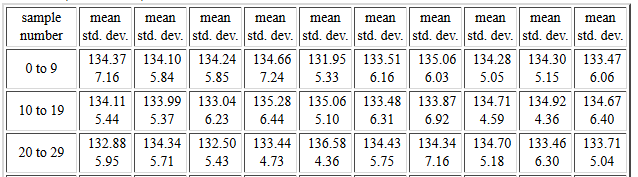After the listing of the 1000 samples, we find in Figure 14 that the report on the samples. One thing to note here is how close the actual standard deviation of the sample means is to the predicted value.

Figure 14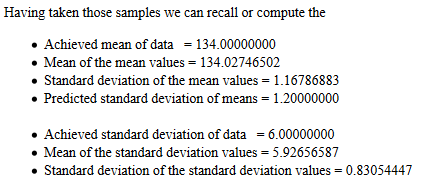The histogram of the 1000 sample means confirms the normal distribution of the sample means.

Figure 15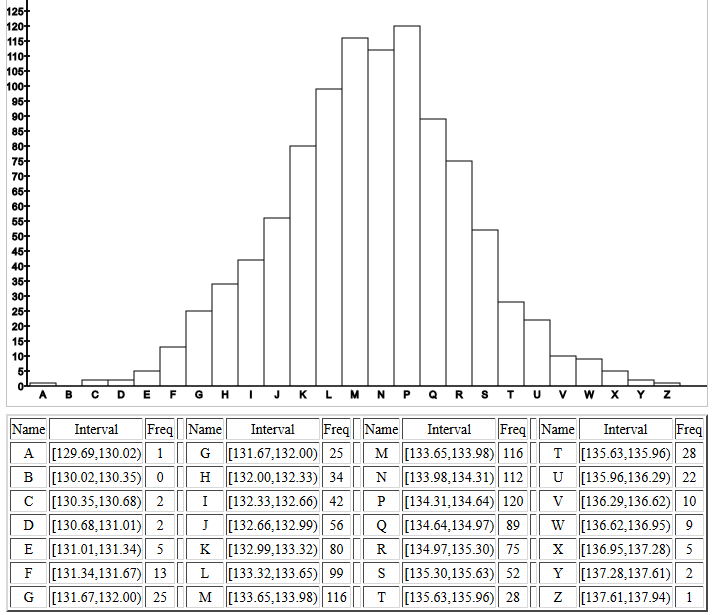Figure 16 shows the start of the listing of the 95% confidence intervals that are generated for each of the samples. The left columns show the construction of the confidence interval based on the known population standard deviation.

The highlighted right columns show the construction of the confidence intervals based on the sample standard deviation and the Student's t distribution. Remember that the confidence level is set to 95% and that the degrees of freedom is 24.

For each sample the table shows the sample mean in the second column, the sample standard deviation in the sixth column, the standard error, which is the sample standard deviation divided by the square root of the sample size, in the seventh column, the margin of error in the eighth column, and the confidence interval in the ninth column.

Figure 16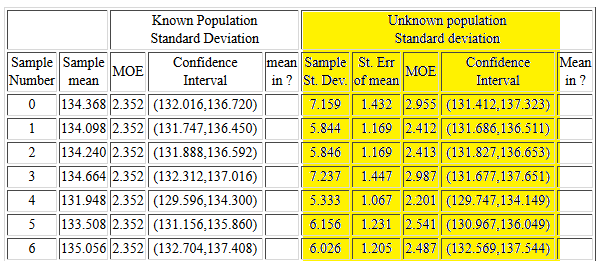There is a lot to learn from the information in that table. First, unlike the confidence intervals on the left where the margin of error is always the same, each of the confidence intervals on the right have different values for the margin of error. Second, the values for the margin of error on the right can be smaller or larger than the uniform value in the left portion. This is true even though the value of tα/2≈-2.064 which is larger than the value of zα/2≈-1.96. This is because the sample standard deviation, sx can be much less than the population standard deviation, σ. For example, look at sample number 4. There the sample standard deviation is 5.333, much less than the population standard deviation, 6. Third, even though the confidence interval on the right can be smaller, in each of the cases shown in Figure 16, both the left and the right confidence intervals contain the true population mean. Remember that we have constructed 95% confidence intervals and therefore we only expect 5% of the intervals that we generate to miss the true population mean. We will have to look down the table to find such examples. Samples 453 through 458 contain such examples. That part of the table is shown in Figure 17.

Figure 17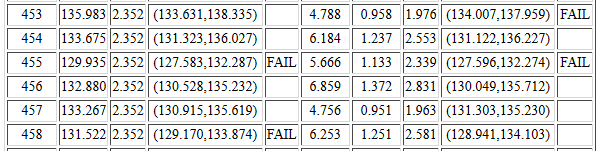For sample #453, if we know the value of σ we generate the left confidence interval and it does contain the true mean. However, sample #453 has a small standard deviation and that, combined with a really high sample mean, gives rise to a narrow confidence interval that does not contain the true mean. That is why the table holds FAIL in the tenth column.

In sample #455 the sample mean is so low that both confidence intervals miss the true μ.

In sample #458 the sample standard deviation is so large that even though the sample has a low mean, the confidence interval constructed using sx still contains μ whereas the confidence interval constructed using σ does not.

At the end of the table of confidence intervals the web page provides the information shown in Figure 18.

Figure 18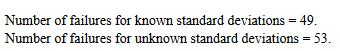From that we learn that 49 of the 1000 confidence intervals generated using σ and the appropriate z-score failed to contain μ. Not bad considering we expect to fail 5% of the 1000 times. We also learn that 53 of the 1000 confidence intervals generated using sx and the appropriate t-score failed to contain μ. Again, not bad considering that we expect to fail 5% of the 1000 times.

#### Automating the process

The process of computing a confidence interval in the case where we do not know the population standard deviation and where we have a sample of size n that yields a sample meanand a sample standard deviation sx is as follows:
1. From the confidence level compute the value of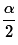using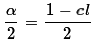2. Use qt() to find the associated t-score, tα/2
3. Find the margin of error as4. Find the two parts to the confidence interval by evaluatingWe should be able to describe these same actions using R statements inside an R function. Consider the following function definition:
```ci_unknown <- function( s=1, n=30,
x_bar=0, cl=0.95)
{
# try to avoid some common errors
if( cl <=0 | cl>=1)
{return("Confidence interval must be strictly between 0.0 and 1")
}
if( s < 0 )
{return("Sample standard deviation must be positive")}
if( n <= 1 )
{return("Sample size needs to be more than 1")}
if( as.integer(n) != n )
{return("Sample size must be a whole number")}
# to get here we have some "reasonable" values
samp_sd <- s/sqrt(n)
t <- abs( qt( (1-cl)/2, n-1))
moe <- t*samp_sd
low_end <- x_bar - moe
high_end <- x_bar + moe
result <- c(low_end, high_end, moe, samp_sd)
names(result)<-c("CI Low","CI High",
"MOE", "Std Error")
return( result )
}
```
This does all of our tasks, including returning the confidence interval as well as some other values. The file of this function is available from ci_unknown.R.

We can try out the new function with the statement ci_unknown(6, 25, 134, 0.95) to do the example we solved back in Figure 4. This is shown in Figure 19.

Figure 19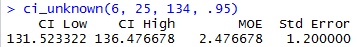To reconstruct the confidence interval for sample #458 shown back in Figure 17 we can use the statement ci_unknown(6.253, 25, 131.522, 0.95) as in Figure 20.

Figure 20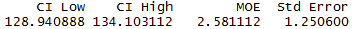#### Worksheet for a Confidence Interval

Here is a link to a worksheet with randomly generated problems related to finding confidence intervals when the standard deviation of the population is unknown.